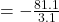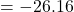## An astronomical telescope has its two lenses spaced 81.1 cm apart. If the objective lens has a focal length of 78.0 cm , what is the magnifi

Question

An astronomical telescope has its two lenses spaced 81.1 cm apart. If the objective lens has a focal length of 78.0 cm , what is the magnification of this telescope? Assume a relaxed eye. Follow the sign conventions.

in progress 0
3 months 2021-08-12T08:03:37+00:00 1 Answers 5 views 0

The magnification is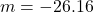Explanation:

From the question we are told that

The distance between the lenses is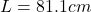The focal length of the objective lens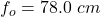Generally the  length of the telescope that satisfies the condition for a relaxed eye can be mathematically represented as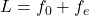Where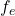is focal length of the eyepiece

Makingthe subject the of the formula above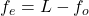Now substituting value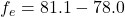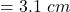Generally magnification can be mathematically represented as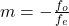This negative sign is a reminder that all real image are always inverted